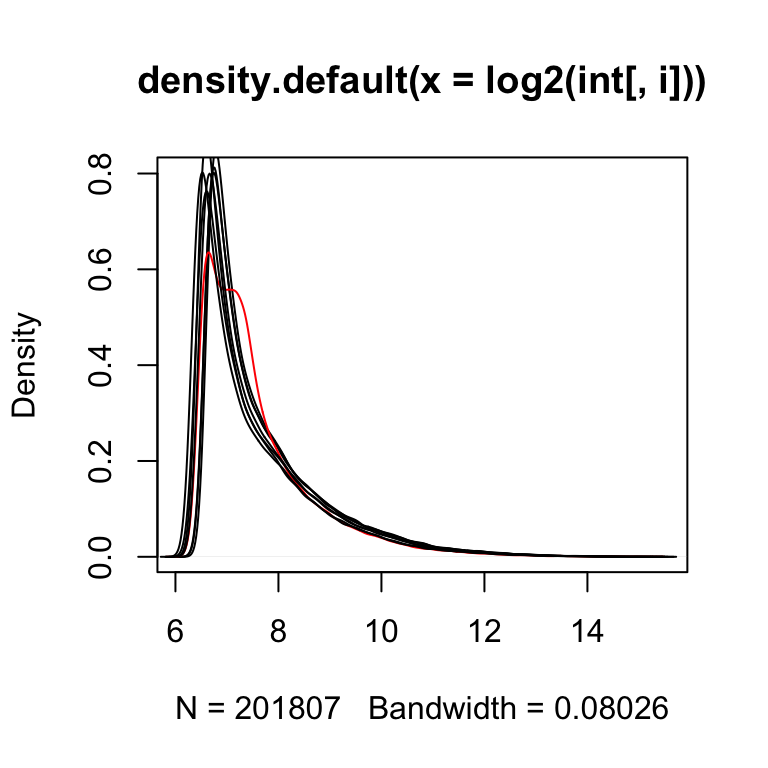# EDA for microarray data

``This analysis was performed using R (ver. 3.1.0).``

## EDA for Microarray data

Exploratory data analysis (EDA) can be used to get an idea of what your data looks like. Here we are analyzing microarray data from 8 samples: two groups of four. A first step in any analysis of genomics data is to learn its general properties and search for problematic samples.

### Histogram

By viewing the data from the first sample we immediately notice that over 90% of data is below 1,000 and the remaining 10% spans values up to 40,000. By taking the log we get a better picture of the distribution. We use base 2 because memorizing the powers of 2 is easy. It gives us a friendly range: 4-16.

``````# library(devtools)
# install_github("dagdata","genomicsclass")
library(dagdata)
data(SpikeInEDA)
#histogramm of the first array: not very informative in linear scale
hist(int[,1])
#Better picture using log2 scale
hist(log2(int[,1]))``````### density curves

Next we look at all eight histograms simultaneously. To facilitate this we introduce the density estimator or smooth histogram. Basically we create a histogram, draw a smooth curve through the top of the bars, and keep that curve. This permits us to put several histograms on the same page:

``````for(i in 1:ncol(int))
if(i==1) plot(density(log2(int[,i])),col=(i==4)+1, main=NULL) else lines(density(log2(int[,i])),col=(i==4)+1)````````Note that one histogram (we highlighted it by making it red) looks different: it has a different shape from the rest. Is this sample different from the rest in any significant way? If we compute the correlation between this sample and the rest it is not very different and all very high.``

### Correlation between samples

``signif(cor(int),2)``
``````##                 2353m99hpp_av08 2353n99hpp_av08 2353o99hpp_av08
## 2353m99hpp_av08            1.00            0.99            0.99
## 2353n99hpp_av08            0.99            1.00            0.99
## 2353o99hpp_av08            0.99            0.99            1.00
## 2353p99hpp_av08            0.99            0.99            0.99
## 2353q99hpp_av08            0.98            0.98            0.98
## 2353r99hpp_av08            0.98            0.98            0.98
## 2353s99hpp_av08            0.98            0.98            0.98
## 2353t99hpp_av08            0.98            0.98            0.98
##                 2353p99hpp_av08 2353q99hpp_av08 2353r99hpp_av08
## 2353m99hpp_av08            0.99            0.98            0.98
## 2353n99hpp_av08            0.99            0.98            0.98
## 2353o99hpp_av08            0.99            0.98            0.98
## 2353p99hpp_av08            1.00            0.98            0.98
## 2353q99hpp_av08            0.98            1.00            0.99
## 2353r99hpp_av08            0.98            0.99            1.00
## 2353s99hpp_av08            0.98            0.99            0.99
## 2353t99hpp_av08            0.98            0.99            0.99
##                 2353s99hpp_av08 2353t99hpp_av08
## 2353m99hpp_av08            0.98            0.98
## 2353n99hpp_av08            0.98            0.98
## 2353o99hpp_av08            0.98            0.98
## 2353p99hpp_av08            0.98            0.98
## 2353q99hpp_av08            0.99            0.99
## 2353r99hpp_av08            0.99            0.99
## 2353s99hpp_av08            1.00            0.99
## 2353t99hpp_av08            0.99            1.00``````

### Scatter plot

The problem is not immediately obvious from a scatter plot.

``````##we don't need to show all the points so we take random samples
#of size 10000
library(rafalib)
splot<-function(x,y,...){
ind<-sample(length(x),10000)
x=x[ind];y=y[ind]
plot(x,y,...)
}
#scatterplot between sample 1 and 2
splot(log2(int[,1]),log2(int[,2]),
main="sample 1 and 2")
#Scatterplot between sample 1 and 4
splot(log2(int[,1]),log2(int[,4]),
main="sample 1 and 4")````````Note that samples 1 through 4 are replicates and should produce the same values up to measurement error. Scatterplots and correlation are not the best tools to detect problems. For example 1,2,3,4 and 100,200,300,400 two lists with very different values have perfect correlation. A better measure is the differences between the values and therefore a better plot is a rotation of the scatter plot containing the differences (log ratios) on the y-axis and the averages (in the log scale) on the x-axis. This plot is a refereed to as an MA-plot (plot of difference versus the average). ``

### MA plot

``````maplot<- function(x,y,...) splot((x+y)/2,y-x,...)
maplot(log2(int[,1]),log2(int[,2]),xlab="A",ylab="M", main="Sple 1 and 2", ylim=c(-2,2))
maplot(log2(int[,1]),log2(int[,3]),xlab="A",ylab="M",main="Sple 1 and 3",ylim=c(-2,2))
maplot(log2(int[,1]),log2(int[,4]),xlab="A",ylab="M", main="Sple 1 and 4", ylim=c(-2,2))````````Now the problem is obvious. It turns out this samples comes from an array for which a spatial problem can be detected at the original image level. We actually have the grid locations for these measurements and can recreate the image.``
``````##we are doing this for two arrays 1 and 4
library(matrixStats) ##need rowMedians
library(RColorBrewer)
for(i in c(1,4)){
r=log2(int[,i])-rowMedians(log2(int))
## r are residuals from median array
## to avoind outliers taking over colors of image
### define a MAX
MAX<-1
r[r>MAX]<-MAX
r[r< -MAX] <- -MAX
##we now that every other column is skipped
mat=matrix(NA,max(locations[,1]),max(locations[,2]+1)/2)
for(j in 1:nrow(locations)){
mat[locations[j,1],(locations[j,2]+1)/2]<-r[j]
}
image(mat,col=brewer.pal(11,"RdBu"))
}````````On the second image we can clearly see the spatial pattern (blue are positive residuals, red are negative)``

Licence